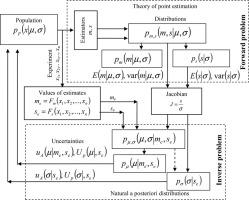Measurement ( IF 3.364 ) Pub Date : 2020-06-27 , DOI: 10.1016/j.measurement.2020.108072
Mykhaylo DorozhovetsIn the article method of Type A uncertainty evaluation of the location and scale parameters of the population based on solution of the forward and inverse problems is proposed. The essence of forward problem is determination of the distributions of estimates m of location and s of scale parameters. The inverse problem is solved when values of the estimates are determined, and its essence is determination of the a posteriori distributions of location and scale parameters and also their standard and expanded uncertainties. A general method of the determining the distributions in inverse problem bases on the transformation of distributions obtained in the forward problem by the Jacobian which is the ratio of estimate (s) to the scale parameter (σ). Independently on population distribution the standard uncertainties of the location and scale parameters are depend on value of 1/sqrt(n − 3), where n is a number of observations.

down
wechat
bug